When creating a Machine Learning or AI application, we always want the most accurate predictions possible. Model optimization can give us better results if we are willing to spend the time. There are many tools and techniques to optimize models for Machine Learning and Artificial Intelligence solutions. One way to optimize your model is to tune the hyperparameters. This can give you a much higher score if done correctly. I will show you how to use 2 of the most popular tools, GridSearchCV and CrossValidation. These tools are very flexible, you can use almost any model in them. I will start with a simple one - RandomForestClassifier. The datasource I will use is an open source dataset containing credit card transactions with fraud/not fraud as the label. This dataset is heavily imbalanced, so I will also include some techniques on how to deal with this complex issue.

In :
# Import Libraries
# try some of these ideas: https://www.tensorflow.org/tutorials/structured_data/imbalanced_data
import numpy as np
import pandas as pd

import os
import time
import matplotlib as mpl
if os.environ.get('DISPLAY','') == '':
print('no display found. Using non-interactive Agg backend')
mpl.use('Agg')

import matplotlib.pyplot as plt
%matplotlib inline
import pandas_profiling as pp
import seaborn as sns

from sklearn.model_selection import KFold
from sklearn.model_selection import GridSearchCV
from sklearn.model_selection import train_test_split
from sklearn.model_selection import cross_val_score
from sklearn.linear_model import LogisticRegression
from sklearn.tree import DecisionTreeClassifier
from sklearn.ensemble import RandomForestClassifier
from sklearn import metrics
from sklearn.metrics import confusion_matrix
from sklearn.metrics import classification_report
from sklearn.metrics import roc_curve
from sklearn.metrics import roc_auc_score
from sklearn.metrics import precision_recall_curve
from sklearn.metrics import f1_score
from sklearn.metrics import auc
from sklearn.metrics import accuracy_score
from sklearn.metrics import precision_score
from sklearn.metrics import recall_score
from sklearn.metrics import cohen_kappa_score
from sklearn.utils.class_weight import compute_sample_weight
from sklearn.preprocessing import StandardScaler
from matplotlib import pyplot
import zipfile

import tensorflow as tf

no display found. Using non-interactive Agg backend

In :
verbose = 0
# alternate location: https://datahub.io/machine-learning/creditcard/r/creditcard.csv

In :
def display_metrics(model_name, train_features, test_features, train_label, test_label, pred):
try:
print(model_name.score(test_features, test_label))
print("Accuracy score (training): {0:.3f}".format(model_name.score(train_features, train_label)))
print("Accuracy score (validation): {0:.3f}".format(model_name.score(test_features, test_label)))
except Exception as e:
print("error")
try:
print(pd.Series(model_name.feature_importances_, index=train_features.columns[:]).nlargest(10).plot(kind='barh'))
except Exception as e:
print("error")

print("Confusion Matrix:")
tn, fp, fn, tp = confusion_matrix(test_label, pred).ravel()
total = tn+ fp+ fn+ tp
print("false positive pct:",(fp/total)*100)
print("tn", " fp", " fn", " tp")
print(tn, fp, fn, tp)
print(confusion_matrix(test_label, pred))
print("Classification Report")
print(classification_report(test_label, pred))
print("Specificity =", tn/(tn+fp))
print("Sensitivity =", tp/(tp+fn))
return tn, fp, fn, tp

In :
def visualize(Actual, Pred, Algo):
#Confusion Matrix
cnf_matrix=metrics.confusion_matrix(Actual, Pred) #

#Visualize confusion matrix using heat map

fig, ax = plt.subplots()
tick_marks = np.arange(len(class_names))
plt.xticks(tick_marks, class_names)
plt.yticks(tick_marks, class_names)

# create heatmap
sns.heatmap(pd.DataFrame(cnf_matrix), annot=True, cmap="YlGnBu" ,fmt='g')
ax.xaxis.set_label_position("top")
plt.tight_layout()
plt.title('Confusion matrix: '+Algo, y=1.1)
plt.ylabel('Actual label')
plt.xlabel('Predicted label')
plt.show()

In :
def auc_roc_metrics(model, test_features, test_labels, algo): # model object, features, actual labels, name of algorithm
# useful for imbalanced data
ns_probs = [0 for _ in range(len(test_labels))]
# predict probabilities
model_probs = model.predict_proba(test_features)
# keep probabilities for the positive outcome only
n = model.predict_proba(test_features).shape-1
model_probs = model_probs[:, n]
model_auc = auc_roc_metrics_plots(model_probs, ns_probs, test_labels, algo)
return model_auc

In :
def auc_roc_metrics_plots(model_probs, ns_probs, test_labels, algo):

# calculate scores
ns_auc = roc_auc_score(test_labels, ns_probs) # no skill
model_auc = round(roc_auc_score(test_labels, model_probs), 4)

# summarize scores
print('%10s : ROC AUC=%.3f' % ('No Skill',ns_auc))
print('%10s : ROC AUC=%.3f' % (algo,model_auc))
# calculate roc curves
ns_fpr, ns_tpr, _ = roc_curve(test_labels, ns_probs)
# NameError: name 'ns_probs' is not defined
model_fpr, model_tpr, _ = roc_curve(test_labels, model_probs)
# plot the roc curve for the model
pyplot.plot(ns_fpr, ns_tpr, linestyle='--', label='No Skill')
pyplot.plot(model_fpr, model_tpr, marker='.', label='%s (area = %0.2f)' % (algo, model_auc))
# axis labels
pyplot.xlabel('False Positive Rate')
pyplot.ylabel('True Positive Rate')
# show the legend
pyplot.legend()
# show the plot
pyplot.show()
return model_auc

In :
def CalcPct(df,title):
unique_elements, counts_elements = np.unique(df, return_counts=True)
calc_pct = round(counts_elements/(counts_elements+counts_elements) * 100,6)
print(title)
print(np.asarray((unique_elements, counts_elements)))
return calc_pct

In :
def plot_loss(history, label, n):
# Use a log scale to show the wide range of values.
plt.semilogy(history.epoch,  history.history['loss'],
color=colors[n], label='Train '+label)
plt.semilogy(history.epoch,  history.history['val_loss'],
color=colors[n], label='Val '+label,
linestyle="--")
plt.xlabel('Epoch')
plt.ylabel('Loss')

plt.legend()

In :
class MyTimer():
def __init__(self):
self.start = time.time()
def __enter__(self):
return self
def __exit__(self, exc_type, exc_val, exc_tb):
end = time.time()
runtime = end - self.start
msg = 'The function took {time} seconds to complete'
print(msg.format(time=runtime))

In :
#try some data cleansing

temp_df = df.copy()
temp_df = temp_df.drop(['Time'], axis=1)
temp_df['Log_Amount'] = np.log(temp_df.pop('Amount')+0.001)
df = temp_df.copy()

In :
X = df.loc[:, df.columns != 'Class']
y = df.loc[:, df.columns == 'Class']
OrigPct = CalcPct(y,"Original")

# split the dataset
# https://scikit-learn.org/stable/modules/generated/sklearn.model_selection.train_test_split.html
test_size = 0.3
val_size = 0.5

class_names=[0,1]

strat = True
if (strat == True):
stratify=y['Class']
else:
stratify="None"
# stratify will ensure that Train, Test and Validation get the same pct of minority classes (.17%)
# X_train, X_test, y_train, y_test = train_test_split(X,y, test_size = 0.3, random_state = None, shuffle=True, stratify=stratify)
X_train, X_test1, y_train, y_test1 = train_test_split(X,y, test_size = test_size, random_state = None, shuffle=True, stratify=stratify)
# then split Test1 into Test and Validate
# Validate will be used as a final benchmark, once all the parameter tuning is completed
X_test, X_val, y_test, y_val = train_test_split(X_test1,y_test1, test_size = val_size, random_state = None, shuffle=True)

TrainPct = CalcPct(y_train,"Train")
TestPct = CalcPct(y_test,"Train")
ValPct = CalcPct(y_val,"Train")
zeros, ones = np.bincount(y_train['Class'])

Original
[[     0      1]
[284315    492]]
Train
[[     0      1]
[199020    344]]
Train
[[    0     1]
[42643    78]]
Train
[[    0     1]
[42652    70]]

In :
# Form np arrays of labels and features for jointplot charts

train_labels = np.array(y_train).flatten()
bool_train_labels = train_labels != 0 # has an extra ,1 in the bool_train_labels.shape
val_labels = np.array(y_val)
test_labels = np.array(y_test)
train_features = np.array(X_train)
val_features = np.array(X_val)
test_features = np.array(X_test)

pos_df = pd.DataFrame(train_features[ bool_train_labels], columns = X.columns)
neg_df = pd.DataFrame(train_features[~bool_train_labels], columns = X.columns)
sns.jointplot(pos_df['V5'], pos_df['V6'],
kind='hex', xlim = (-5,5), ylim = (-5,5))
plt.suptitle("Positive distribution")
sns.jointplot(neg_df['V5'], neg_df['V6'],
kind='hex', xlim = (-5,5), ylim = (-5,5))
_ = plt.suptitle("Negative distribution")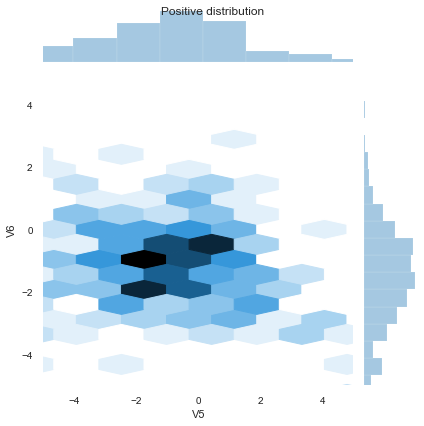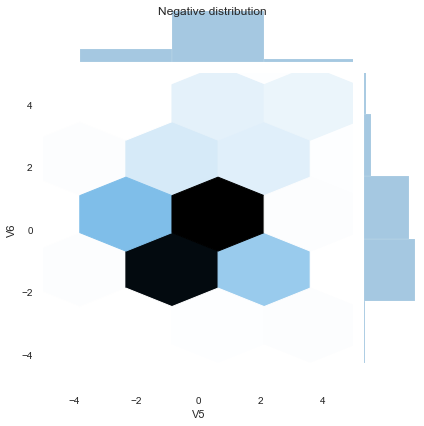In :
# find the number of minority (value=1) samples in our train set so we can down-sample our majority to it
yes = len(y_train[y_train['Class'] ==1])

# retrieve the indices of the minority and majority samples
yes_ind = y_train[y_train['Class'] == 1].index
no_ind = y_train[y_train['Class'] == 0].index

# random sample the majority indices based on the amount of
# minority samples
new_no_ind = np.random.choice(no_ind, yes, replace = False)

# merge the two indices together
undersample_ind = np.concatenate([new_no_ind, yes_ind])

# get undersampled dataframe from the merged indices of the train dataset
X_train = X_train.loc[undersample_ind]
y_train = y_train.loc[undersample_ind]

In :
sc = StandardScaler()
X_train = sc.fit_transform(X_train)
X_test = sc.transform(X_test)
X_val = sc.transform(X_val)

# handle any extreme fliers, set to 5 or -5
X_train = np.clip(X_train, -5, 5)
X_test = np.clip(X_test, -5, 5)
X_val = np.clip(X_val, -5, 5)

In :
y_train = np.array(y_train).flatten()
rf = RandomForestClassifier(n_estimators = 1000)

print("Fitting First Draft Model with Train data and default parameters:")
with MyTimer():
rf.fit(X_train, y_train)

print(rf.score(X_train, y_train))

rf.get_params

Fitting First Draft Model with Train data and default parameters:
The function took 3.5789904594421387 seconds to complete
1.0

Out:
<bound method BaseEstimator.get_params of RandomForestClassifier(bootstrap=True, ccp_alpha=0.0, class_weight=None,
criterion='gini', max_depth=None, max_features='auto',
max_leaf_nodes=None, max_samples=None,
min_impurity_decrease=0.0, min_impurity_split=None,
min_samples_leaf=1, min_samples_split=2,
min_weight_fraction_leaf=0.0, n_estimators=1000,
n_jobs=None, oob_score=False, random_state=None,
verbose=0, warm_start=False)>

K-Folds cross-validator

Provides train/test indices to split data in train/test sets. Split dataset into k consecutive folds (without shuffling by default). Each fold is then used once as a validation while the k - 1 remaining folds form the training set.

https://scikit-learn.org/stable/auto_examples/model_selection/plot_nested_cross_validation_iris.html

Nested versus non-nested cross-validation This example compares non-nested and nested cross-validation strategies on a classifier of the iris data set. Nested cross-validation (CV) is often used to train a model in which hyperparameters also need to be optimized. Nested CV estimates the generalization error of the underlying model and its (hyper)parameter search. Choosing the parameters that maximize non-nested CV biases the model to the dataset, yielding an overly-optimistic score.

Model selection without nested CV uses the same data to tune model parameters and evaluate model performance. Information may thus “leak” into the model and overfit the data. The magnitude of this effect is primarily dependent on the size of the dataset and the stability of the model. See Cawley and Talbot 1 for an analysis of these issues.

To avoid this problem, nested CV effectively uses a series of train/validation/test set splits. In the inner loop (here executed by GridSearchCV), the score is approximately maximized by fitting a model to each training set, and then directly maximized in selecting (hyper)parameters over the validation set. In the outer loop (here in cross_val_score), generalization error is estimated by averaging test set scores over several dataset splits.

The example below uses a support vector classifier with a non-linear kernel to build a model with optimized hyperparameters by grid search. We compare the performance of non-nested and nested CV strategies by taking the difference between their scores.

In :
param_grid={
'n_estimators': range(50,126,25), # number of trees
'class_weight':['balanced',None],
'max_samples':[0.2,0.4,0.6,0.8],
#'max_features': range(50,401,50),
#'max_features': [50,100], # can be list or range or other
'max_features':[None,'sqrt','log2'],
'min_samples_leaf': range(10,40,10),
'min_samples_split': range(20,80,10),
'criterion':['gini','entropy']
}

# Number of random trials to use for KFold Strategy
NUM_TRIALS = 2

# Arrays to store scores
non_nested_scores = np.zeros(NUM_TRIALS)
nested_scores = np.zeros(NUM_TRIALS)

# Loop for each trial
for i in range(NUM_TRIALS):

# To be used within GridSearch
inner_cv = KFold(n_splits=2, shuffle=True, random_state=None)

# To be used in outer CV
outer_cv = KFold(n_splits=2, shuffle=True, random_state=None)

#inner loop KFold example:
gsc = GridSearchCV(
estimator=rf,
param_grid=param_grid,
scoring='roc_auc', # or 'r2', etc
#scoring='neg_mean_squared_error', # or look here for other choices
# https://scikit-learn.org/stable/modules/model_evaluation.html#scoring-parameter
#cv=5,
cv=inner_cv, # this will use KFold splitting to change train/test/validation datasets randomly
verbose=verbose
)

print("Running GridSearchCV:")
with MyTimer():
grid_result = gsc.fit(X_train, y_train)
non_nested_scores[i] = grid_result.best_score_

print("Best: %f using %s" % (grid_result.best_score_, grid_result.best_params_))

# nested/non-nested cross validation: https://scikit-learn.org/stable/auto_examples/model_selection/plot_nested_cross_validation_iris.html
with MyTimer():
nested_score = cross_val_score(gsc, X=X_train, y=y_train, cv=outer_cv, verbose=verbose).mean()
# source code for cross_val_score is here: https://github.com/scikit-learn/scikit-learn/blob/master/sklearn/model_selection/_validation.py#L137
print("nested score from KFold")
print(nested_score)
nested_scores[i] = nested_score

if (verbose == 1):
#for test_mean, train_mean, param in zip(
for test_mean, param in zip(
grid_result.cv_results_['mean_test_score'],
#grid_result.cv_results_['mean_train_score'],
grid_result.cv_results_['params']):
print("Test : %f with: %r" % (test_mean, param))

score_difference = non_nested_scores - nested_scores

print("Average difference of {:6f} with std. dev. of {:6f}."
.format(score_difference.mean(), score_difference.std()))

Running GridSearchCV:
The function took 1267.9618706703186 seconds to complete
Best: 0.982515 using {'class_weight': 'balanced', 'criterion': 'gini', 'max_features': None, 'max_samples': 0.2, 'min_samples_leaf': 30, 'min_samples_split': 20, 'n_estimators': 75}
The function took 2026.9131433963776 seconds to complete
nested score from KFold
0.9737092391304348
Running GridSearchCV:
The function took 1156.3172867298126 seconds to complete
Best: 0.982074 using {'class_weight': None, 'criterion': 'entropy', 'max_features': None, 'max_samples': 0.6, 'min_samples_leaf': 10, 'min_samples_split': 20, 'n_estimators': 50}
The function took 2088.7608046531677 seconds to complete
nested score from KFold
0.9742757664875097
Average difference of 0.008302 with std. dev. of 0.000504.

In :
wt1 = 4.0
wt0 = 1.0
# create your optimized best model using the best params from CV
rf = RandomForestClassifier(**grid_result.best_params_)

# fit the model you created with the best settings earlier
print("Fitting Model (Train) with GridSearch and Cross Validation optimized params:")
#rf.fit(X_train, y_train)
rf.fit(X_train, y_train, sample_weight=np.where(y_train == 1, wt1, wt0).flatten())

print("Train rf.score")
print(rf.score(X_train, y_train))

Fitting Model (Train) with GridSearch and Cross Validation optimized params:
Train rf.score
0.9578488372093024

In :
# Plot scores on each trial for nested and non-nested CV
plt.figure()
plt.subplot(211)
non_nested_scores_line, = plt.plot(non_nested_scores, color='r')
nested_line, = plt.plot(nested_scores, color='b')
plt.ylabel("score", fontsize="14")
plt.legend([non_nested_scores_line, nested_line],
["Non-Nested CV", "Nested CV"],
bbox_to_anchor=(0, .4, .5, 0))
plt.title("Non-Nested and Nested Cross Validation on Credit Card Fraud Dataset",
x=.5, y=1.1, fontsize="15")

# Plot bar chart of the difference.
plt.subplot(212)
difference_plot = plt.bar(range(NUM_TRIALS), score_difference)
plt.xlabel("Individual Trial #")
plt.legend([difference_plot],
["Non-Nested CV - Nested CV Score"],
bbox_to_anchor=(0, 1, .8, 0))
plt.ylabel("score difference", fontsize="14")

plt.show()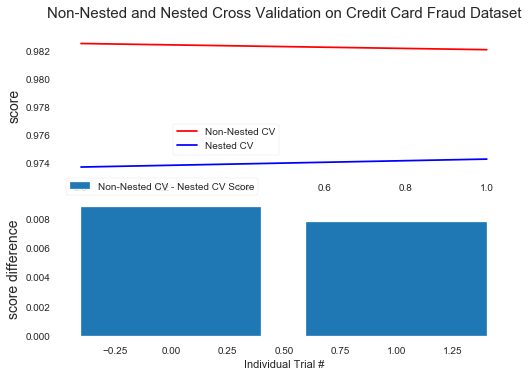In :
print("True Model Score from Test Data:")
print(rf.score(X_test, y_test))

True Model Score from Test Data:
0.9154046019522015


Validate the grid parameters used in my model

In :
grid_result

Out:
GridSearchCV(cv=KFold(n_splits=2, random_state=None, shuffle=True),
error_score=nan,
estimator=RandomForestClassifier(bootstrap=True, ccp_alpha=0.0,
class_weight=None,
criterion='gini', max_depth=None,
max_features='auto',
max_leaf_nodes=None,
max_samples=None,
min_impurity_decrease=0.0,
min_impurity_split=None,
min_samples_leaf=1,
min_samples_split=2,
min_weight_...
iid='deprecated', n_jobs=None,
param_grid={'class_weight': ['balanced', None],
'criterion': ['gini', 'entropy'],
'max_features': [None, 'sqrt', 'log2'],
'max_samples': [0.2, 0.4, 0.6, 0.8],
'min_samples_leaf': range(10, 40, 10),
'min_samples_split': range(20, 80, 10),
'n_estimators': range(50, 126, 25)},
pre_dispatch='2*n_jobs', refit=True, return_train_score=False,
scoring='roc_auc', verbose=0)

Show the results of the GridSearchCV function

In :
# show results of the GridSearch
print('wt1', 'wt0')
print(wt1, wt0)
print("Best: %f using %s" % (grid_result.best_score_, grid_result.best_params_))

wt1 wt0
4.0 1.0
Best: 0.982074 using {'class_weight': None, 'criterion': 'entropy', 'max_features': None, 'max_samples': 0.6, 'min_samples_leaf': 10, 'min_samples_split': 20, 'n_estimators': 50}


Use this model to predict the Test and Validate datasets

In :
# use this model to predict a new dataset
rf_Pred=rf.predict(X_test)

#print(metrics.accuracy_score(y_test, y_pred))
#print(classification_report(y_test, rf_Pred))
print("Test Data results")
tn, fp, fn, tp = display_metrics(rf, X_train, X_test, y_train, y_test, rf_Pred)
#visualize(y_test, rf_Pred, 'RF')
rf_auc = auc_roc_metrics(rf, X_test, y_test, 'RF')
print('rf_auc', rf_auc)

# use this model to predict a new dataset
rf_Pred=rf.predict(X_val)

#print(metrics.accuracy_score(y_val, y_pred))
#print(classification_report(y_val, rf_Pred))
print("\nVal Data results")
tn, fp, fn, tp = display_metrics(rf, X_train, X_val, y_train, y_val, rf_Pred)
#visualize(y_val, rf_Pred, 'RF')
rf_auc = auc_roc_metrics(rf, X_val, y_val, 'RF')
print('rf_auc', rf_auc)

Test Data results
0.9154046019522015
Accuracy score (training): 0.958
Accuracy score (validation): 0.915
error
Confusion Matrix:
false positive pct: 8.440813651365838
tn  fp  fn  tp
39037 3606 8 70
[[39037  3606]
[    8    70]]
Classification Report
precision    recall  f1-score   support

0       1.00      0.92      0.96     42643
1       0.02      0.90      0.04        78

accuracy                           0.92     42721
macro avg       0.51      0.91      0.50     42721
weighted avg       1.00      0.92      0.95     42721

Specificity = 0.9154374692212086
Sensitivity = 0.8974358974358975
No Skill : ROC AUC=0.500
RF : ROC AUC=0.975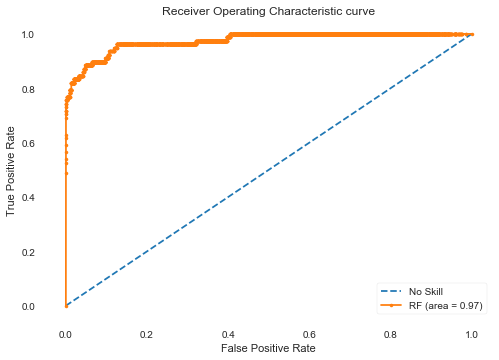rf_auc 0.9746

Val Data results
0.9125743176817565
Accuracy score (training): 0.958
Accuracy score (validation): 0.913
error
Confusion Matrix:
false positive pct: 8.73320537428023
tn  fp  fn  tp
38921 3731 4 66
[[38921  3731]
[    4    66]]
Classification Report
precision    recall  f1-score   support

0       1.00      0.91      0.95     42652
1       0.02      0.94      0.03        70

accuracy                           0.91     42722
macro avg       0.51      0.93      0.49     42722
weighted avg       1.00      0.91      0.95     42722

Specificity = 0.9125246178373816
Sensitivity = 0.9428571428571428
No Skill : ROC AUC=0.500
RF : ROC AUC=0.981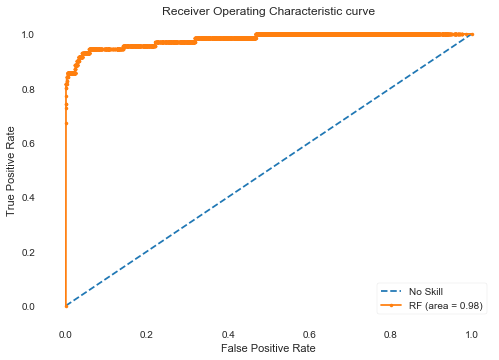rf_auc 0.9805

In [ ]: